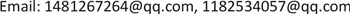1重庆交通大学土木工程学院，重庆

2温州市铁路与轨道交通投资集团有限公司，浙江 温州

3浙江大学宁波理工学院，土木建筑工程学院，浙江 宁波1. 引言

2. 数值计算模型及材料参数2.1. 几何模型

2.2. 材料参数2.2.1. 炸药材料模型

p 1 = A 1 ( 1 − ω R 1 V ) e − R 1 V + B 1 ( 1 − ω R 2 V ) e − R 2 V + ω E 0 V (1)

2.2.2. 药型罩的属性参数

σ y = ( A + B ε ¯ p n ) ( 1 + c ln ε ˙ ∗ ) ( 1 − T * m ) (2)

ε ¯ p ：有效塑性应变； ε ∗ = ε ¯ p ∗ / ε 0 ∗ ， ε 0 ∗ = 1   s − 1 时的有效塑性应变率；A、B、C、n、m是和材料性质有关的常数。(2)式中 T ∗ 为：

T ∗ = ( T − T r ) / ( T m − T r ) (3)

ε f = [ D 1 + D 2 exp D 3 σ ∗ ] [ 1 + D 4 ln ε ∗ ] [ 1 + D 5 T * ] (4)

σ ∗ = σ / σ e f f (5)

D = ∑ Δ ε ¯ p ε f (6)

The main parameter value of the copper-shaped hood Johnson-Cook material model (g-cm-μs-k

p = ρ 0 C 1 2 μ [ 1 + ( 1 − γ 0 2 ) μ − a 2 μ 2 ] [ 1 − ( S 1 − 1 ) μ − S 2 μ 2 μ + 1 − S 3 μ 3 ( μ + 1 ) 2 ] + ( γ 0 + α μ ) E (7)

p = ρ 0 C 1 2 μ + ( γ 0 + α μ ) E (8)

Parameter values of the Grüneisen equation for the copper coating (g-cm-μs-k

2.2.3. 岩石的基本物理参数

Basic physical parameters of granite (Micro-weathering, Kv = 0.85, Kf = 0.80

Basic physical parameters of granite (Moderate weathering, Kv = 0.60, Kf = 0.40

2.3. 有限元模型

3. 射孔弹成型聚能射流特性分析

4. 射孔弹侵彻岩石数值分析

5. 结论

1) 确定了数值计算中所采用的炸药、金属药型罩、微风化以及中风化花岗岩石的理论模型及材料参数，为准确描述岩石损伤特征规律奠定了良好的基础。

2) 建立射孔弹与岩石相互作用的有限元计算模型，初步确定将射孔弹爆炸后成型的聚能射流与爆炸场的耦合毁伤效应作为对岩石最佳毁伤模式的数值计算研究方向。

3) 针对射孔弹成型聚能射流的三段式(药型罩压垮变形阶段、射流稳定成型阶段以及射流拉伸断裂阶段)典型特征分析，获得射流成型过程中不同阶段对岩石毁伤的规律特征。

4) 以微风化花岗岩石为作用目标，结合射孔弹成型聚能射流与岩石作用的毁伤规律特征确定了适合射孔弹对岩石毁伤的工况特征，即当射流处于稳定成型阶段时射流自身具有较高的动能适合对岩石进行较大深度的侵彻且此时射孔弹炸药爆炸产生的爆炸场也能够有效增加对岩石内部结构的毁伤，起到增大射孔弹穿深以及增加岩石内部裂纹数量的毁伤效果，从而有效达到工程应用的目标。